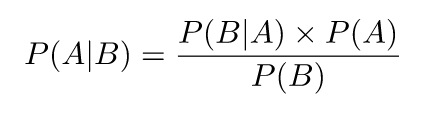# Naïve Bayes Explained with Course Ratings Prediction Example in PythonNaïve Bayes Explained with Course Ratings Prediction Example in Python

## Introduction to Naïve Bayes

### Bayes’ TheoremImage screenshot by Americana

Bayes’ Theorem is a mathematical formula that allows us to calculate the ‘reversed’ conditional probabilities. It is often used when we have some prior belief about the probability of an event happening, and want to incorporate that information to calculate the conditional probability of an outcome. We could define the terms as follows:

• P(A|B) is referred to as the posterior probability
• P(A) is the prior probability
• P(B|A) is the likelihood
• P(B) is the evidence.
• We can then restate the formula as :

Posterior = Likelihood * Prior / Evidence

### Naïve Bayes

• Given a set of data {(x1,y1) …… (xn,yn)}
• y takes a limited set of discrete values( categories) from 1 to k

Naïve Bayes applies the concept of Bayes Theorem to compute P(yi=1|xi), P(yi=2|xi) …. P(yi=k|xi) *from the value of *P(xi|yi=1), P(xi|yi=2)….P(xi|yi=k) and the value of P(x) & P(y=1)….P(y=k)

Our prediction is simply the y value that gives the maximum among all P(yi=1|xi), P(yi=2|xi) …. P(yi=k|xi).

## Top Machine Learning Projects in Python For Beginners  | upGrad blog

If you want to become a machine learning professional, you’d have to gain experience using its technologies and also by completing projects. Top Machine Learning Projects in Python For Beginners 

## Top Machine Learning Projects in Python For Beginners 

If you want to become a machine learning professional, you’d have to gain experience using its technologies. The best way to do so is by completing projects. Take a look at this article and we will help you become an expert

## Start a Career in Machine Learning and Artificial Intelligence

Enroll now at best Artificial Intelligence training in Noida, - the best Institute in India for Artificial Intelligence Online Training Course and Certification.

## How are deep learning, artificial intelligence and machine learning related

What is the difference between machine learning and artificial intelligence and deep learning? Supervised learning is best for classification and regressions Machine Learning models. You can read more about them in this article.

## AI(Artificial Intelligence): The Business Benefits of Machine Learning

Enroll now at CETPA, the best Institute in India for Artificial Intelligence Online Training Course and Certification for students & working professionals & avail 50% instant discount.